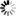Welcome to Central Library, SUST
Normal view

# A Logical Introduction to Proof [electronic resource] / by Daniel W. Cunningham.

Material type:TextPublisher: New York, NY : Springer New York : Imprint: Springer, 2013Description: XVI, 356 p. online resourceContent type:
• text
Media type:
• computer
Carrier type:
• online resource
ISBN:
• 9781461436317
Additional physical formats: Printed edition:: No titleDDC classification:
• 510 23
LOC classification:
• QA1-939
Online resources:
Contents:
Preface -- The Greek Alphabet -- 1. Propositional Logic -- 2. Predicate Logic -- 3. Proof Strategies and Diagrams -- 4. Mathematical Induction -- 5. Set Theory -- 6. Functions -- 7. Relations -- 8. Core Concepts in Abstract Algebra -- 9. Core Concepts in Real Analysis -- A Summary of Strategies -- References -- List of Symbols. Index.
Summary: A Logical Introduction to Proof is a unique textbook that uses a logic-first approach to train and guide undergraduates through a transition or “bridge” course  between calculus and advanced mathematics courses.  The author’s approach  prepares the student for the rigors required in future mathematics courses and is appropriate for majors in mathematics, computer science, engineering, as well as other applied mathematical sciences. It may also be beneficial as a supplement for students at the graduate level who need guidance or reference for writing proofs.   Core topics covered are logic, sets, relations, functions, and induction, where logic is the instrument for analyzing the structure of mathematical assertions and is a tool for composing mathematical proofs. Exercises are given at the end of each section within a chapter. Chapter 1 focuses on propositional logic while Chapter 2 is devoted to the logic of quantifiers. Chapter 3 methodically presents the key strategies that are used in mathematical proofs; each presented as a proof diagram. Every proof strategy is carefully illustrated by a variety of mathematical theorems concerning the natural, rational, and real numbers. Chapter 4 focuses on mathematical induction and concludes with a proof of the fundamental theorem of arithmetic. Chapters 5 through 7 introduce students to the essential concepts that appear in all branches of mathematics. Chapter 8 introduces the basic structures of abstract algebra: groups, rings, quotient groups, and quotient rings. Finally, Chapter 9 presents proof strategies that explicitly show students how to deal with the fundamental definitions that they will encounter in real analysis, followed by numerous examples of proofs that use these strategies.  The appendix provides a useful summary of strategies for dealing with proofs.
Tags from this library: No tags from this library for this title.
Star ratingsAverage rating: 0.0 (0 votes)
No physical items for this record

Preface -- The Greek Alphabet -- 1. Propositional Logic -- 2. Predicate Logic -- 3. Proof Strategies and Diagrams -- 4. Mathematical Induction -- 5. Set Theory -- 6. Functions -- 7. Relations -- 8. Core Concepts in Abstract Algebra -- 9. Core Concepts in Real Analysis -- A Summary of Strategies -- References -- List of Symbols. Index.

A Logical Introduction to Proof is a unique textbook that uses a logic-first approach to train and guide undergraduates through a transition or “bridge” course  between calculus and advanced mathematics courses.  The author’s approach  prepares the student for the rigors required in future mathematics courses and is appropriate for majors in mathematics, computer science, engineering, as well as other applied mathematical sciences. It may also be beneficial as a supplement for students at the graduate level who need guidance or reference for writing proofs.   Core topics covered are logic, sets, relations, functions, and induction, where logic is the instrument for analyzing the structure of mathematical assertions and is a tool for composing mathematical proofs. Exercises are given at the end of each section within a chapter. Chapter 1 focuses on propositional logic while Chapter 2 is devoted to the logic of quantifiers. Chapter 3 methodically presents the key strategies that are used in mathematical proofs; each presented as a proof diagram. Every proof strategy is carefully illustrated by a variety of mathematical theorems concerning the natural, rational, and real numbers. Chapter 4 focuses on mathematical induction and concludes with a proof of the fundamental theorem of arithmetic. Chapters 5 through 7 introduce students to the essential concepts that appear in all branches of mathematics. Chapter 8 introduces the basic structures of abstract algebra: groups, rings, quotient groups, and quotient rings. Finally, Chapter 9 presents proof strategies that explicitly show students how to deal with the fundamental definitions that they will encounter in real analysis, followed by numerous examples of proofs that use these strategies.  The appendix provides a useful summary of strategies for dealing with proofs.

There are no comments on this title.